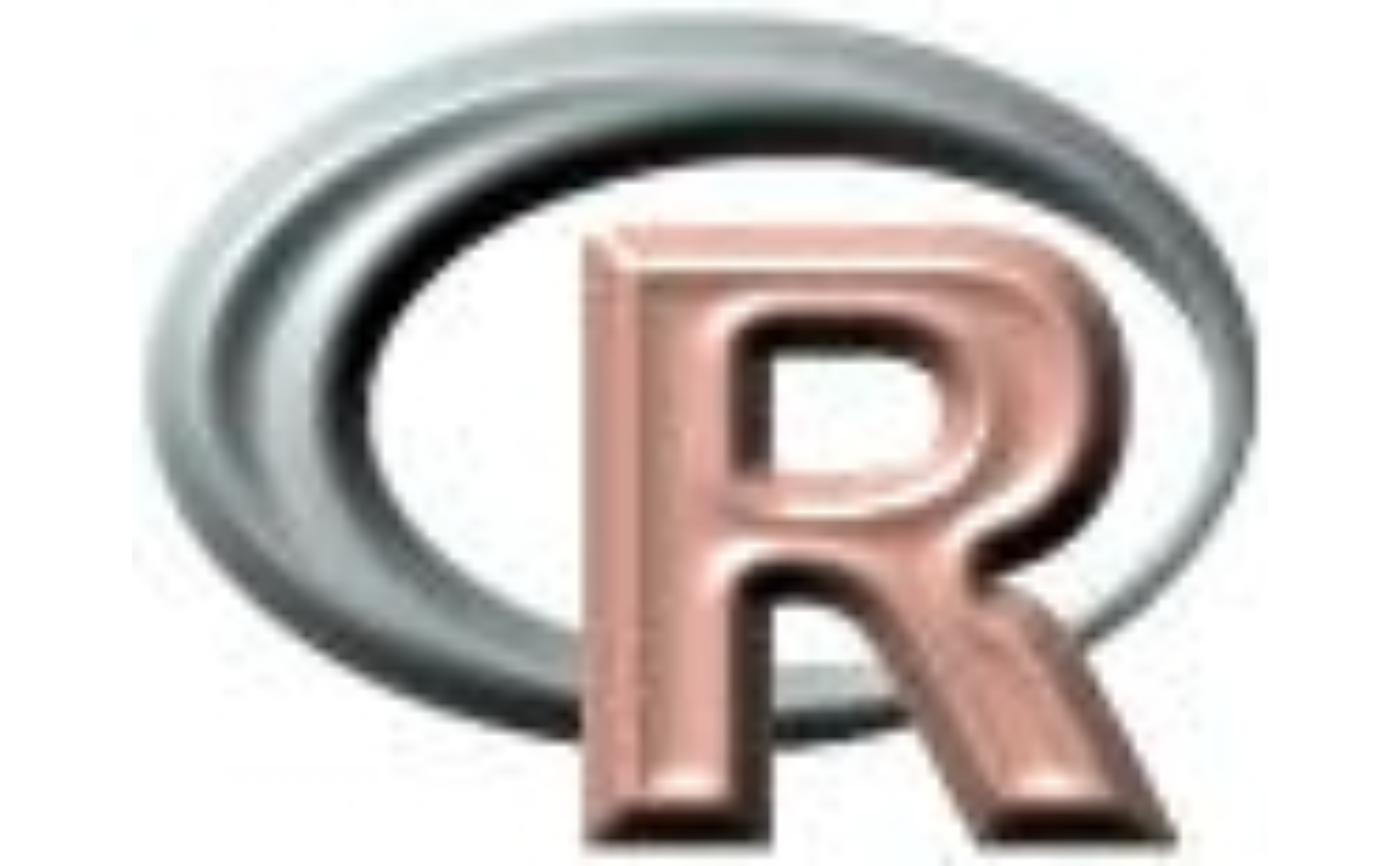Make a Red-Green-Blue plot based on three layers in a SpatRaster. The layers (sometimes referred to as "bands" because they may represent different bandwidths in the electromagnetic spectrum) are combined such that they represent the red, green and blue channel. This function can be used to make "true" (or "false") color images from Landsat and other multi-spectral satellite images.

Note that the margins of the plot are set to zero (no axes or titles are visible) but can be set with the mar argument.

An alternative way to plot RGB images is to first use colorize to create a single layer SpatRaster with a color-table and then use plot.

# S4 method for SpatRaster
plotRGB(x, r=1, g=2, b=3, a=NULL, scale=NULL, mar=0,
stretch=NULL, smooth=TRUE, colNA="white", alpha=NULL, bgalpha=NULL,
zlim=NULL, zcol=FALSE, axes=FALSE ,...)

## Arguments

x

SpatRaster

r

integer between 1 and nlyr(x). Layer to use as the Red channel

g

integer between 1 and nlyr(x). Layer to use as the Green channel

b

integer between 1 and nlyr(x). Layer to use as the Blue channel

a

NULL or integer between 1 and nlyr(x). Layer to use as the alpha (transparency) channel. If not NULL, argument alpha is ignored

scale

integer. Maximum (possible) value in the three channels. Defaults to 255 or to the maximum value of x if that is known and larger than 255

mar

numeric vector recycled to length 4 to set the margins of the plot. Use mar=NULL or mar=NA to not set the margins

stretch

character. Option to stretch the values to increase contrast: "lin" (linear) or "hist" (histogram)

smooth

logical. If TRUE, smooth the image when drawing to get the appearance of a higher spatial resolution

colNA

color. The color used for cells that have NA values

alpha

transparency. Integer between 0 (transparent) and 255 (opaque)

bgalpha

Background transparency. Integer between 0 (transparent) and 255 (opaque)

zlim

numeric vector of length 2. Range of values to plot (optional). If this is set, and stretch="lin" is used, then the values are stretched within the range of zlim. This allows creating consistent coloring between SpatRasters with different cell-value ranges, even when stretching the colors for improved contrast

zcol

logical. If TRUE the values outside the range of zlim get the color of the extremes of the range. Otherwise, the values outside the zlim range get the color of NA values (see argument "colNA")

axes

logical. If TRUE axes are drawn (and arguments such as main="title" will be honored)

...

graphical parameters as in plot<SpatRaster-method>

plot, colorize, RGB

## Examples

b <- rast(system.file("ex/logo.tif", package="terra"))
plotRGB(b)
plotRGB(b, mar=2)plotRGB(b, 3, 2, 1)b[1000:2000] <- NA
plotRGB(b, 3, 2, 1, stretch="hist")